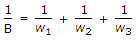# Electronics and Communication Engineering - Analog Electronics

36.

In a practical oscillator, the magnitude of loop gain Aβ is kept slightly larger than unity and the amplitude of oscillations is limited by

 A. the ratio of A to β B. the on set of non-linearity of amplifying device C. the linearity of amplifying device D. all of the above

Explanation:

No answer description available for this question. Let us discuss.

37.

Assertion (A): Direct coupled amplifiers are rarely used

Reason (R): Direct coupled amplifiers suffer from the disadvantage of drift

 A. Both A and R are correct and R is correct explanation for A B. Both A and R are correct but R is not correct explanation for A C. A is correct R is wrong D. A is wrong R is correct

Explanation:

No answer description available for this question. Let us discuss.

38.

A multistage amplifier has a low pass response with three real poles at s = - w1 - w2 and w3. The approximate overall bandwidth B of the amplifier will be given by

 A. B = w1 + w2 + w3 B.C. B = (w1 + w2 + w3)1/3 D. B = w12 + w22 + w32

Explanation:

No answer description available for this question. Let us discuss.

39.

In a feedback series regular circuit, the output voltage is regulated by controlling the

 A. magnitude of the I/P voltage B. gain of the feed back BJT C. reference Voltage D. voltage drop across the series pass transistor

Explanation:

No answer description available for this question. Let us discuss.

40.

The transformer cores operating at microwave frequency ranges are generally made up of

 A. silicon steel B. ferrites C. albino D. super alloy